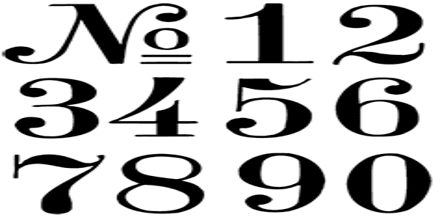Mathematic

# Lecture on NumbersPrime objective of this lecture is to present on Numbers. Here briefly describe various terms of numbers. Perfect Square: Squares of whole numbers; Square root: One of two equal factors of a number. If a squared equals b then a is the square root of b. The square root of 144 is 12 because 12 squared is 144. here also focus on Radical Sign: The sign used to represent a non-negative square root. Irrational Number: Numbers that cannot be expressed as a/b. Real Number: Irrational numbers together with rational numbers to form the set of all numbers.